## Monday, July 29, 2019

When I first started using R, my code looked just like MATLAB code. I read an intro to R programming, did some data analysis using R, and I was using the arrow assignment operator! But otherwise, my R code looked very much like MATLAB. Then I read Advanced R by Hadley Wickham. I found out, while I wasn't doing anything wrong, I wasn't getting the most out of R.

Advanced R is very readable if you've used R and maybe some other programming experience. If you're self-taught in R and have no other programming experience, you may find the book a bit tough. The book does a great job describing the important features and how to make the most of them. I particularly liked the chapter on functions and the section on functional programming.

I highly recommend it!

https://www.amazon.com/dp/1466586966/ref=cm_sw_su_dp?tag=devtools-20

Update: A commenters pointed out there is a new version of the book out. The link is below.

## Wednesday, June 26, 2019

### Blackman-Tukey Spectral Estimator in R

Blackman-Tukey Spectral Estimator in R!

There are two definitions of the power spectral density (PSD). Both definitions are mathematically nearly identical and define a function that describes the distribution of power over the frequency components in our data set. The periodogram PSD estimator is based on the first definition of the PSD (see periodogram post). The Blackman-Tukey spectral estimator (BTSE) is based on the second definition. The second definition says, find the PSD by calculating the Fourier transform of the autocorrelation sequence (ACS). In R this definition is written as

```PSD <- function(rxx) {
fft(rxx)
}
```

where fft is the R implementation of the fast Fourier transform, rxx is the autocorrelation sequence (ACS), the k'th element of the ACS rxx[k] = E[xx[k]], k -infinity to +infinity, and E is the expectation operator. The xx in rxx[k] is a reminder r is a correlation between x and itself. The rxx[k]s are sometimes called lags. The ACS has the propriety that rxx[-k]=rxx[k]*, where * is the complex conjugate. In the post, we will only use real numbers and I'll drop the * from here forward.

So, to find the PSD we just calculate rxx and take its fft! Unfortunately, in practice, we cannot do this. Calculating the expected value requires the probability density function (PDF) of x, which we don't know and we need an infinite amount of data, which we don't have. So, we can't calculate the PSD: we're doomed!

No, we are not doomed. We can't calculate the PSD, but we estimate it! We can derive an estimator for the PSD from the definition of the PSD. First, we replace rxx with an estimate of rxx. We replace the expected value, which gives the exact rxx, with an average, which gives us an estimate of rxx. The E[xx[k]] is replaced with (1/N)(xx[1+k]+xx[2+k]+...+x[N-1-k]x[N-1]+x[N-k]x[N]), where N is the number of data samples. For example; if k=0, then rxx[k]=(1/N)*sum(x*x). In R code the estimate is written as

```lagEstimate <-function(x,k,N=length(x)){
(1/N)*sum(x[1:(N-k)]*x[(k+1):N])
}
```

If we had an infinite amount of data, N=infinity, we could use lagEstimate to estimate the entire infinite ACS. Unfortunately we don't have an infinite amount of data, even if we did it wouldn't fit into a computer. So, we can only estimate a finite amount of ASC elements. The function below calculates lags 0 to kMax.

```Lags <-function(x,kMax) {
sapply(0:kMax,lagEstimate,x=x)
}
```

Before we can try these functions out we need data. In this case the data came from a random process with the PSD plotted in the figure below. The x-axis is normalized frequency(frequency divided by the sampling rate). So, if the sampling rate was 1000 Hz, you could multiply the normalized frequency by 1000 Hz and then the frequency axis would read 0 Hz to 1000 Hz. The y-axis in in dB (10log10(amplitude)). You can see six large sharp peaks in the plot and a gradual dip towards 0 Hz and then back up. Some of the peaks are close together and will be hard to resolve.The data produced by the random process is plotted below. This is the data we will use through this post. The data is posted here: x.RDataLet's calculate the the ACS up to the 5th lag using the data.

```Lags(x,kMax=5)
```
```##   6.095786 -1.368336  3.341608  1.738122 -1.737459  3.651765
```

A kMax of 5 gives us 6 lags: {r, r, r, r, r, r}. These 6 lags are not an ACS, but are part of an ACS.

We used Lags to estimate the positive lags up to kMax, but the ACS is even sequence, r[-k]=r[k] for all k. So, let's write a function to make a sequence consisting of lags from r[-kMax] to r[kMax]. This is a windowed ACS, values outside of the +/- kMax are replaced with 0. Where it won't cause confusion, I'll refer to the windowed ACS, as the ACS.

```acsWindowed <- function(x,kMax,Nzero=0){
rHalf <- c(Lags(x,kMax),rep(0,Nzero))
c(rev(rHalf[2:length(rHalf)]),rHalf)
}
```

Let's try this function out.

```acsW <- acsWindowed(x,9)
```

In the figure below you can see the r lag, the maximum, is plotted in the middle of the plot.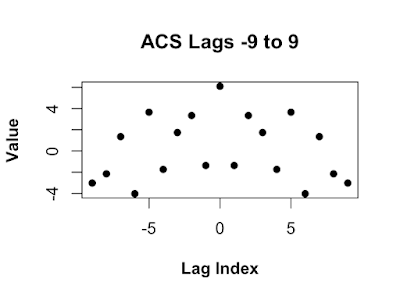The ACS in the figure above is how the ACS is usually plotted in textbooks. In textbooks the sum in the Fourier transform ranges from -N/2 to (N-1)/2. So, the r lag should be in the center of the plot. In R the sum in the Fourier transform ranges from 1 to N, so the 0'th lag has to be first. We could just make the sequence in R form, but it is often handy to start in textbook from and switch to R form. We can write a function to make switching from textbook to R easy.

```Textbook2R <- function(x,N=length(x),foldN=ceiling(N/2)) {
c(x[foldN:N],x[1:(foldN-1)])
}
```

Notice in the figure below the maximum lag r, is plotted at the beginning.Let's imagine we have an infinite amount of data and used it to estimated and infinite number of ACS lags. Let's call that sequence rAll. We make a windowed ACS by setting rW=rAll*W, where W=1 for our 9 lags and 0 everywhere else. W is called the rectangular window, because, as you can see in the plot below, it's plot looks like a rectangle. By default when we estimate a finite number of lags we are using a rectangular window.

```W <- c(rep(0,9),rep(1,9),rep(0,9))
```The reason we can not use a rectangular window is its Fourier Transform is not always positive. As you can see in the plot below there are several values below zero, indicated with dotted line. Re() functions removes some small imaginary numbers due to numerical error, some imaginary dust we have to sweep up.

```FFT_W <- Re(fft(Textbook2R(W)))
```Even though the fft of the ACS rAll is positive , the produce rAll and a rectangular window might not be positive! The Bartlett window is a simple window whos fft is positive.

```BartlettWindow <- function(N,n=seq(0, (N-1)))  {
1 - abs( (n-(N-1)/2) / ( (N-1)/2) )
}
Wb <- BartlettWindow(19)
```

As you can see in the plot below the Fourier transform of the Bartlett window is positive.

```WbFft <- Re(fft(Textbook2R(Wb)))
```## Calculating the BTSE with R

Now that we can estimate the ACS and window our estimate, we are ready to estimate the PSD of our data. The BTSE is written as

```Btse <- function(rHat,Wb) {
Re(fft(rHat*Wb))
}
```

Note the Re() is correcting for numerical error.

In the first example we use a 19 point ACS lag sequence.

```rHat   <- Textbook2R(acsWindowed(x,kMax=9))
Wb     <- Textbook2R(BartlettWindow(length(rHat)))
Pbtse9 <- Btse(rHat,Wb)
```

In the figure below is the BTSE calculated with a maximum lag of 9. The dotted lines indicate the locations of the peaks in the PSD we are trying to estimate. The estimate with a maximum lage of only 9 produces a poor estimate.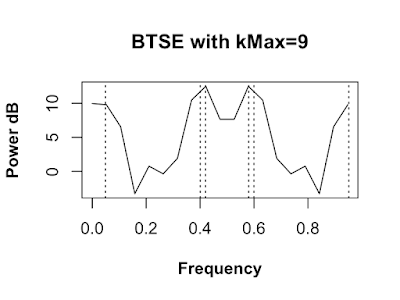We calculate a new estimate with a maximum lag of 18.

```rHat    <- Textbook2R(acsWindowed(x,kMax=18))
Wb      <- Textbook2R(BartlettWindow(length(rHat)))
Pbtse18 <- Btse(rHat,Wb)
```

The next estimate is made with a maximum lag of 18. This estimate is better, the peaks around 0.4 and 0.6 are not resolved. We still need to increase the maximum lag.Finally we increase the maximum lag to 65 and recalculate the estimate.

```rHat    <- Textbook2R(acsWindowed(x,kMax=65))
Wb      <- Textbook2R(BartlettWindow(length(rHat)))
Pbtse65 <- Btse(rHat,Wb)
```

This finial estimate is very good. All six peaks are resolved and the location of our estimated peaks are very close to the true peak locations locations.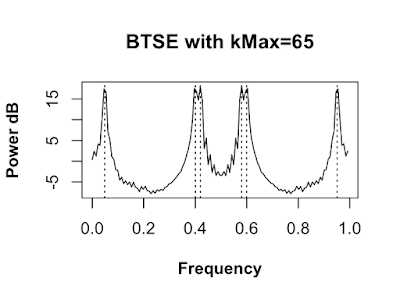## Final Thoughts

Could we use 500 lags in the BTSE? In this case we could, since we have a lot of data, but the higher lags get estimated with less data and therefore have more variance. Using the high variance lags will produce a higher variance estimate.

Are there other ways to improve the BTSE other than using more lags? Yes! There are a few other ways. For instance, we could zero pad the lags. Basically add zeros to the end of our lag sequence. This will make the fft, in the BTSE estimator, evaluate the estimate at more frequencies and we will be able to see more details in the estimated PSD.

Also keep in mind there are other PSD estimation methods that do better on other PSD features. For instance, if you we more interested finding deep nulls rather than peaks, a moving average PSD estimation would be better.

## Thursday, June 13, 2019

### Periodogram with R

Periodogram with R
The power spectral density (PSD) is a function that describes the distribution of power over the frequency components composing our data set. If we knew the process that generated the data, we could just calculate the PSD; we would not have to estimate it. Unfortunately, in practice we won't have access to the random process, only the samples (data) produced by the process. So, we can't get the true PSD, we can only get an estimate of the true PSD. In our example below, we made the data, so we know what the true PSD should look like.
The PSD is written as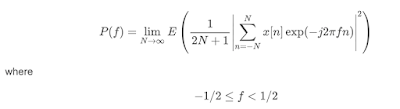where -1/2 <= f < 1/2 x is the data, e is the complex exponential, the sum of the data x multiplied by e is the Fourier transform. In English, calculate the PSD by finding the expected value of the magnitude squared of the Fourier transform as the amount of data increases to infinity. Well, that's a mouthful! The frequency f is expressed in normalized frequency. Normalized frequency is the frequency divided by the sampling rate. Normalized frequency is often used for simplicity. To convert to frequency in Hz multiply by the sampling rate.
The periodogram estimator is based on the definition in equation above. The definition has a limit and an expected value. To use the definition directly we would need an infinite amount of data, which we don't have, and we would need to know the probability density function (PDF) of the data which we don't know. To use this definition to get an estimator of the PSD, we must drop the limit and we must drop the expectation operator (\$E\$). We also can only evaluate a finite number of frequencies. After dropping the limit and the expectation we are left withwhere 0 <= f < 1 and N is the number of data samples. Notice, the range of f changes from (-1/2,1/2) in the first equation to (0,1) in the second equation. Textbooks usually use the former and R uses the latter.
The periodogram is very easy to implement in R, but before we do we need to simulate some data. The code below first uses the set.seed command so R will produce the same "random" numbers each time. Then it creates a 32 normally distributed numbers and 32 points of a sine wave with a normalized frequency of 0.4 and a amplitude of 2. The signal is made up of a sine wave and the random points added together.
The figure below is a plot of the data generated above. To me it looks random and it is not obvious there is a sine wave in there.
```set.seed(0)
N <-32
n<- 0:(N-1)
w <- rnorm(1:N)
f1 <- 0.4
A1 <- 2
s <- A1*sin(2*pi*f1*n)
x <- s + w
```
Now we are going to implement the periodogram in R. It is pretty much a direct translation from the math. We start with the 1/N and multiply that by the absolute value of the square of the Fourier transform of the data. Writing that in English was more work than implementing the periodogram in R!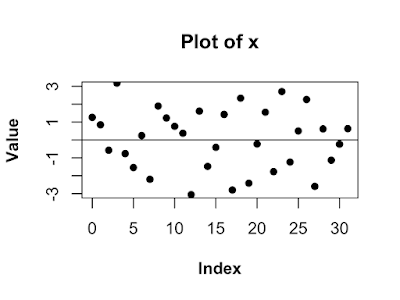```xPer <- (1/N)*abs(fft(x)^2)
f    <- seq(0,1.0-1/N,by=1/N)
```
The figure below is a plot of the periodogram of the data. The dotted line marks the location of the frequency f1, the frequency of the sine wave of the data. Now the sine wave component really stands out!. Notice two things. First, the peaks of the periodogram seems to be a bit off the true values. Second, the plot looks jagged. Both of these things are caused by the same thing: the periodogram is only evaluated at 32 frequency bins. The frequencies we evaluate the periodogram on are called bins. We can fix both problems by evaluating the periodogram at more bins. One way to evaluate the periodogram at more points is to get more data! That would certainly fix both problems. Unfortunately, we often are struck with the data we have. There is another way.Since f is a continuous variable we can evaluate the periodogram at as many points as we want. The way we do this is to pretend we have more data by sticking zeros at the end of our data, we zero pad it. The R code below adds 968 zeros to the end of x zero-padding it to a total of 1000 "data" points. As you can see in the figure below, we have fixed both problems. In the 32 point periodogram missed the peak, because the periodogram was not evaluated at enough points. The zero-padding does not guarantee we hit the peak, but it will get closer. Also, we fixed the jaggedness by evaluating the periodogram at many more bins.
```xzp <- c(x,rep(0,1000-N))
Nzp <- length(xzp)
```
```xPerZp <- (1/N)*abs(fft(xzp)^2)
fzp    <- seq(0,1.0-1/Nzp,by=1/Nzp)
```## Monday, June 3, 2019

### JavaScript is Fast?

JavaScript is Fast?

OK, I admit it. I'm always looking for the one true programming language. Mostly I use programming languages for signal processing, data science, that kind of thing. So, for me I want a language to be good for prototyping, flexible, fast, have lots of math/statistics libraries. The first language I use for signal processing was C, not much to say about that. I then became a heavy MATLAB user, great for a lot of things. I used MathCad. It was great for making readable code! I even used Mathematica. I never got the hang of it's syntax, it always felt award. Lately, I've been doing most of my stuff in R.

So, I find myself at the Julia site, at the time is was the next new mathematical programming language. They have a benchmark page showing how fast it is and it is fast! On that page they show JavaScript as faster than a bunch of standard math mathematically oriented languages. I would not have guessed that. Maybe, JavaScript should be the shiny "new" programming language for math!

## Sunday, June 2, 2019

### What is a Statistic?

What is a Statistic?

A statistic is a mathematical operation on a data set, performed to get information from the data.

Below is the R code that generates 20 random samples. The samples are uniformly distributed between 0 and 1. Uniformly means all the data samples are equally likely, like when you flip a coin heads and tails are equally likely.

```x <- round(runif(20),2)
x
```
```##   0.90 0.27 0.37 0.57 0.91 0.20 0.90 0.94 0.66 0.63 0.06 0.21 0.18
##  0.69 0.38 0.77 0.50 0.72 0.99 0.38
```

One thing we might want to know about this data set it the expected value (EV). The EV is the typical value of the data. Since we know the data is uniformly distributed between 0 and 1, the EV is just the middle of the range, 0.5. In practice, we have the data, but don't know the distribution the data came from. So, we can't calculate the EV, but we could use the average as an estimate of the EV. The R code below is the average.

```sum(x)/20
```
```##  0.5615
```

The average of the data is about 0.56. The average is not the only way to estimate the EV, there are many ways! We could just use the first element of the data set 0.90 as the estimate. Another way is to find the maximum of the data and divide it by 2. The code below finds the max of the data and divides it by 2. Notice the max/2 produces an estimate that is closer to the true value of 0.5 than the average.

```max(x)/2
```
```##  0.495
```

There are many way to estimate the EV, but some are better than others. Estimators of random data are also random. So, the only way to compare estimators is statistically. The case above where the max/2 produced a better estimate than the average could have been luck. The code below generates 20 data samples from the same uniform distribution above 20 times. We then find the estimate the EV in three ways: the average, the first sample, and the max(x)/2.

```trials <- 100
avg     <- matrix(0,1,trials)
first   <- matrix(0,1,trials)
halfMax <- matrix(0,1,trials)
for (indx in 1:trials) {
x <- runif(20)
avg[indx]     <- sum(x)/20
first[indx]   <- x
halfMax[indx] <- max(x)/2
}
```

The figure below contains 100 averages plotted with circles and true EV plotted in a line at 0.5. Most of the averages are between 0.4 and 0.6 and many are closer. The average seems to be a good estimate of the EV. Which shouldn't be surprising since the average is the most common statistic.This figure is the estimate based only on the first element of the data set. The estimates are between just over 0 and almost 1. A few estimates are close to 0.5, but most are not. This is not a good estimator for EV. This is probably not surprising since this estimate is based on only one sample and the average is based on 20.This figure is the plot of the estimate based on the max/2. Most of the estimates are very close to the true value. It looks even better than the average! This may seem surprising, since it looks like it is based on only one sample, the maximum sample, but it is actually makes use of all the samples. This is because you need to look at all the samples to know which one is the maximum.The average is a good choice (and often the best choice) for estimating the EV, but it is not always the best estimator for EV. In the case of this particular distribution, the max/2 is better estimator of EV than the average.

So, we now know what a statistic is and we worked with a few. As a field of study, statistics uses visualization, probability and other math tools to find the best ways to get information from data.

## Monday, May 27, 2019

### Book Review: Data Analysis with Open Source Tools

This book gives a very good overview of the kinds of things data scientists do and the tools they use.  All the concepts are illustrated with hands on programing examples you can follow along with.

I think this book would be very good for two groups of people. First, someone working in a technical field who wants to know what data science is all about. This would give them a good idea of the skills they will need and the type of work they may do as a data scientist.

The second group that would benefit from this book are people engaged in some field of data science and who are looking for a broad overview of the field. Since many people came to data science from other fields and learned hands on they may have trouble seeing the forest for the trees.

This is not a pop science book. If you looking for an armchairs introduction to the wonderful world of data science, this is not the book for you. If you want to learn by doing and know a bit of math and have some familiarity with programing, then this is the book for you.

https://www.amazon.com/Data-Analysis-Source-Tools-Hands/dp/0596802358

## Sunday, May 26, 2019

### Mirror image data with MATLAB

A sequence is even if x[midpoint-n] = x[midpoint+n] for some midpoint and for all n. There are many cases where it is handy to have a even sequence. For Instance, the Fourier transform of an even sequence is real and it is handy to have a real function in the transform domain.

Below is the some MATLAB code that will make a sequence even by appending a mirror image section. The function will mirror image one, two and three dimensional data.

function DataMirrorImage = MirrorImageData(Data)
% This function mirror images one, two and three dimensional data in all
% in one, two or three dimensions.
%
% INPUT:
% Data3D................The data to be mirror imaged.
%
% OUTPUT:
% DataMirrorImage.....The mirror image data.

% Get the dimensions of the data.
[xLen, yLen, zLen] = size(Data);

% Mirror image the data in 1D or 2D.
for zNdx = 1:zLen
Data2D = squeeze(Data(:,:,zNdx));
Data2D = [ Data2D fliplr(Data2D(:,2:yLen))];
Data2DMirrorImage = [ Data2D; flipud(Data2D(2:xLen,:))];
DataMirrorImage(:,:,zNdx) = Data2DMirrorImage;
end

% If data is 3D , mirror image the data in 3D.
if zLen > 1
Data3DMirrorImage(:,:,1:zLen) = DataMirrorImage;
Data3DMirrorImage(:,:,(zLen+1):(2*zLen-1)) = flipdim(DataMirrorImage(:,:,2:zLen),3);
DataMirrorImage = Data3DMirrorImage;
end

Example:

>> x = randn(1,9)
x =
1.4172    0.6715   -1.2075    0.7172    1.6302    0.4889    1.0347    0.7269   -0.3034
>> xMi = MirrorImageData(x)
xMi =
Columns 1 through 10
1.4172    0.6715   -1.2075    0.7172    1.6302    0.4889    1.0347    0.7269   -0.3034   -0.3034
Columns 11 through 17
0.7269    1.0347    0.4889    1.6302    0.7172   -1.2075    0.6715

>> x = randn(9);
>> imagesc(x)

>> imagesc(MirrorImageData(x))

## Saturday, May 25, 2019

### 'Semi-infinite' Sounds like a lot!

Looks like we'll be making computers for a while. https://www.independent.co.uk/environment/rare-earth-metals-japan-semi-infinite-ocean-mobile-phones-electric-cars-a8301966.html

## Friday, May 24, 2019

### Random Autocorrelation Sequences R version

Random Autocorrelation Sequences R version

### What is an autocorrelation sequence?

Autocorrelation sequences (ACSs) are super common when doing anything in probability and statistics. Autocorrelation is a sequence of measurements of how similar a sequence is to it self. In math the autocorrelation sequence r[k] is

r[k] = E[x[n]x[n+k]] for k={0,1,...N-1},

where N is the number of data samples, E is the expected value, x[n] is a data sample and k is the lag. The lag is the separation in samples.

### Why make a random autocorrelation sequence?

When testing an algorithm or conducting simulations it is often useful to use a random ACS. Generating random a random ACS can be difficult because they have a lot of special properties and if you select a sequence at random, the chance it is a valid ACS is small.

### Trick to making a random autocorrelation sequence

We can use the following property of ACSs to make generating random ACSs easy. The ACS and the power spectral density (PSD) are Fourier transform (FT) pairs. For our purpose here, a PSD is just a function that is positive everywhere. "FT pair" means the FT of an ACS is a PSD and the inverse FT of a PSD is an ACS.

So we can generate a random ACS using the following steps. First, generate a random sequence. Second, square each element, so the sequence is positive. Finally, find the inverse FT of the squared sequence.

### The R code that produces a random ACS

The ACS could be any size, but in this case we want a 9 element sequence.

N <- 9
PSD <- rnorm(N)^2
ACS <- fft(PSD,inverse = TRUE)

The line below outputs the ACS and as you can see it is a complex sequence.

ACS

 0.6183715+0.0000000i -0.1375219+0.1960568i -0.1672163-0.2084656i 0.2199730-0.0977208i
 -0.0281983+0.2615475i -0.0281983-0.2615475i 0.2199730+0.0977208i -0.1672163+0.2084656i
 -0.1375219-0.1960568i

### What if I want a real ACS

If you want a real ACS then the PSD has to be even. So, let's make the sequence even!

PSDeven <- c(PSD,PSD[N:2])
PSDeven

 0.39244438 0.03372487 0.69827518 2.54492084 0.10857537 0.67316837 0.23758708 0.54512337
 0.33152416 0.33152416 0.54512337 0.23758708 0.67316837 0.10857537 2.54492084 0.69827518
 0.03372487

Notice the ACS is still complex. Numerical error causes some imaginary dust we need to clean up.

ACS <- fft(PSDeven,inverse = TRUE)/N
ACS

 1.1931381+0i 0.1714080+0i -0.3200109+0i -0.5007558+0i -0.2372697+0i 0.2647028+0i
 0.4700409+0i 0.3009945+0i -0.3750372+0i -0.3750372+0i 0.3009945+0i 0.4700409+0i
 0.2647028+0i -0.2372697+0i -0.5007558+0i -0.3200109+0i 0.1714080+0i

Clean up the small imaginary part with Re() and now we are ready to plot.

ACS <- Re(ACS)

### Plot of ACS

The figure below is a plot of the ACS from lag k = 0 to 16. In textbooks the ACS would have been plotted from k=-8 to 8, with r in the center.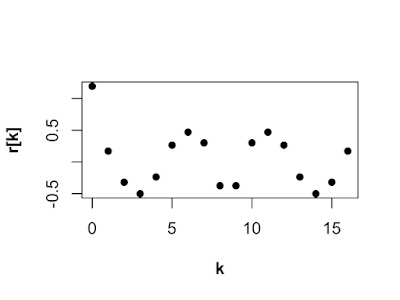This is the plot of the ACS in the textbook style. Notice, the lag at 0 r is positive and larger than the other lags, a standard property of ACSs. All is well!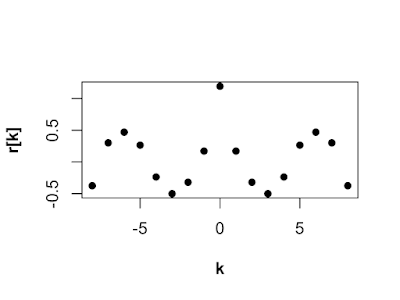## Thursday, May 23, 2019

This is a generated video of someone talking made from one  image!!

https://youtu.be/p1b5aiTrGzY?t=47

## Wednesday, May 22, 2019

### Random Autocorrelation Sequences MATLAB version

Autocorrelation sequences (ACSs) are commonly used in a variety of fields. When testing an algorithm or conduction simulations it is sometimes useful to use a random ACS. Generating random a random ACS can be difficult because they have a lot of special properties and if you select a sequence at random, the chance it is a valid ACS is small.

We can use the following property of ACSs to make generating random ACSs easy. The ACS and the power spectral density (PSD) are Fourier transform (FT) pairs. For our purpose here, a PSD is just a function that is positive everywhere. "FT pair" means the FT of an ACS is a PSD and the invers FT of a PSD is an ACS.

So we can generate a random ACS using the following steps. First, generate a random sequence. Second, square each element. Finally, find the inverse FT of the squared sequence.

The matlab code below does just that.
N = 9;
PSD = randn(1,N).^2;
ACS = ifft(PSD);
ACS
ACS =
1.0022 + 0.0000i  -0.0828 + 0.1658i   0.1847 - 0.0446i   0.0978 + 0.1778i   0.3034 - 0.3709i   0.3034 + 0.3709i   0.0978 - 0.1778i   0.1847 + 0.0446i  -0.0828 - 0.1658i